# Test: Pie Chart - 1

## 10 Questions MCQ Test Topic-wise Past Year Questions for CAT | Test: Pie Chart - 1

Description
Attempt Test: Pie Chart - 1 | 10 questions in 15 minutes | Mock test for CAT preparation | Free important questions MCQ to study Topic-wise Past Year Questions for CAT for CAT Exam | Download free PDF with solutions
QUESTION: 1

### Directions for Questions: Answer the questions on the basis of the information given below. Pie chart – 1 givens the percentage shares of all the five cement companies –A, B, C, D and E – in the total quantity of cement sold in country XYZ. Pie Chart 2 givens the percentage shares of all the eleven states – P through Z – in the total quantity of cement sold in the country.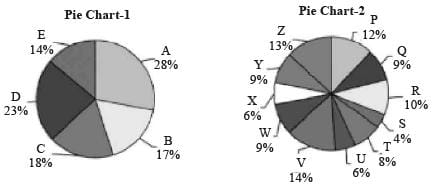The market share of any company in a state is the total quantity of cement sold by the company in that state as a percentage of the total quantity of cement sold in that state. (2016) Q. In any state, if no company had more than 50% market share, then in at least how many states did company A sell cement?

Solution:

To find the least number of states in which company A sold cement, we have to assume that company A had its sales in states in which the total sales were the maximum. Even if we assume company A had 50% market share in the states with maximum sales, there must be at least 5 states where company A sold cement.

QUESTION: 2

### Directions for Questions: Answer the questions on the basis of the information given below. Pie chart – 1 givens the percentage shares of all the five cement companies –A, B, C, D and E – in the total quantity of cement sold in country XYZ. Pie Chart 2 givens the percentage shares of all the eleven states – P through Z – in the total quantity of cement sold in the country.The market share of any company in a state is the total quantity of cement sold by the company in that state as a percentage of the total quantity of cement sold in that state. (2016) Q. If in all the states in which company E was present, it had a market share of at least 25%, in at most how many states did company E sell cement?

Solution:

To find the maximum number of states where company E was present, we have to assume it had sales in the states where the total sales are minimum and it had a share of only 25% in the states.
∴ Company E had its sales in at most 7 states (S, U, X, T, Q, W and Y). It cannot include R also as 25% of sales in R is 2.5% of total and already at least 25% of 51% = 12.75% is accounted by the seven states.
According tograph, only one company ie (A) which had sales in more than two states.

QUESTION: 3

### Directions for Questions: Answer the questions on the basis of the information given below. Pie chart – 1 givens the percentage shares of all the five cement companies –A, B, C, D and E – in the total quantity of cement sold in country XYZ. Pie Chart 2 givens the percentage shares of all the eleven states – P through Z – in the total quantity of cement sold in the country.The market share of any company in a state is the total quantity of cement sold by the company in that state as a percentage of the total quantity of cement sold in that state. (2016) Q. The number of companies which had sales in more than two states, is at least

Solution:
QUESTION: 4

Directions for Questions: Answer the questions on the basis of the information given below.
The pie chart given below shows the break-up of production cost of six products – A through F – of Zen Private Ltd. in year 2011.
The total production cost was ₹ 250 Cr.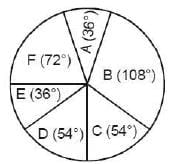Each of the six products is produced in two varieties- Type P and Type Q. The ratio of the units produced for each product and the profit percentage on selling them is given in the table below.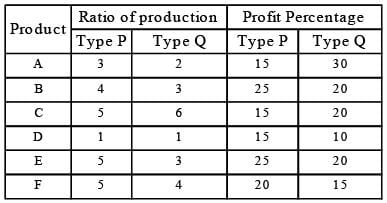Also for each product, the cost of production per item of  Type P and Type Q varieties are in the ratio 4 : 5.

(2015)

Q. For how many of the six products, is the profit made on items of type Q not more than the profit made on items of type P?

Solution: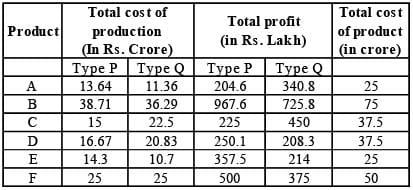(B, D, E, F) Only four products – B, D, E and F, are the profit made on items of type Q not more than the profit made on item of type P.

QUESTION: 5

Directions for Questions: Answer the questions on the basis of the information given below.
The pie chart given below shows the break-up of production cost of six products – A through F – of Zen Private Ltd. in year 2011.
The total production cost was ₹ 250 Cr.Each of the six products is produced in two varieties- Type P and Type Q. The ratio of the units produced for each product and the profit percentage on selling them is given in the table below.Also for each product, the cost of production per item of  Type P and Type Q varieties are in the ratio 4 : 5.

(2015)

Q. For which product is the ratio of total profit to total production cost, the lowest?

Solution: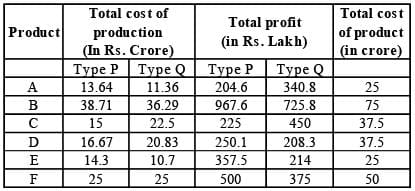According to above data, product D is the ratio of total profit is total productive on cost is the 10 lowest.

QUESTION: 6

Directions for Questions: Answer the questions on the basis of the information given below.
The pie chart given below shows the break-up of production cost of six products – A through F – of Zen Private Ltd. in year 2011.
The total production cost was ₹ 250 Cr.Each of the six products is produced in two varieties- Type P and Type Q. The ratio of the units produced for each product and the profit percentage on selling them is given in the table below.Also for each product, the cost of production per item of  Type P and Type Q varieties are in the ratio 4 : 5.

(2015)

Q. For how many products, overall profit percentage is more than 20%?

Solution:A, B and E overall profit percentage is more than 26%.

QUESTION: 7

Directions for Questions: Answer the questions on the basis of the information given below.
The pie chart given below shows the break-up of production cost of six products – A through F – of Zen Private Ltd. in year 2011.
The total production cost was ₹ 250 Cr.Each of the six products is produced in two varieties- Type P and Type Q. The ratio of the units produced for each product and the profit percentage on selling them is given in the table below.Also for each product, the cost of production per item of  Type P and Type Q varieties are in the ratio 4 : 5.

(2015)

Q. The nearest integer to the total cost (In ₹Cr.) incurred in producing type A of products A, D & F is

Solution:Total Cost inurred in producing type P of products A, D & F = 13.64 + 16.67 + 25 = ₹ 55.31 crore.

QUESTION: 8

Directions for Questions: Answer the questions on the basis of the information given below.
The following pie chart gives details of the production, expenses and export of the six products manufactured by company KL Enterprises, which manufactures only the given six products, in the year 2014. In the given year, the company followed a very strict internal audit policy and any item that did not meet the specifications were rejected and disposed off. All the products exported were those manufactured in the same year itself.

(2015)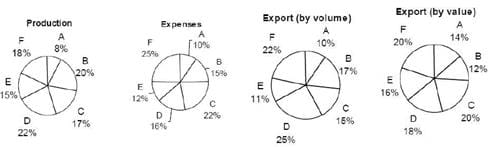Profit = Sales – Expenses
Profitability(%) = Profit / Expenses x 100

Q. In 2014, the products exported as a percentage of the products manufactured by the company could not be more than

Solution:

Let the production be 100x and export (volume) be 100y As the share in export for three products (i.e. A, D and F is more than that in production but A witness the maximum change in share.)
For maximizing the export (volume) assume all volume of A is exported
∴ 8x = 10y ⇒ y / x = 0.08 = 80%

QUESTION: 9

Directions for Questions: Answer the questions on the basis of the information given below.
The following pie chart gives details of the production, expenses and export of the six products manufactured by company KL Enterprises, which manufactures only the given six products, in the year 2014. In the given year, the company followed a very strict internal audit policy and any item that did not meet the specifications were rejected and disposed off. All the products exported were those manufactured in the same year itself.

(2015)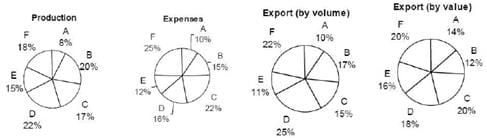Profit = Sales – Expenses
Profitability(%) = Profit / Expenses x 100

Q. In 2014, if product D was a profit making product for the company, what was the maximum number of products that were loss making products for the company?

Solution:

Let the expenses and export by value be 100 x and 100y respectively.
As D is making profit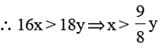QUESTION: 10

Directions for Questions: Answer the questions on the basis of the information given below.
The following pie chart gives details of the production, expenses and export of the six products manufactured by company KL Enterprises, which manufactures only the given six products, in the year 2014. In the given year, the company followed a very strict internal audit policy and any item that did not meet the specifications were rejected and disposed off. All the products exported were those manufactured in the same year itself.

(2015)Profit = Sales – Expenses
Profitability(%) = Profit / Expenses x 100

Q. The export price per unit of which product was the highest?

Solution:

Let export (by volume) and export by value be 100x and 100y respectively then price per unit for all products is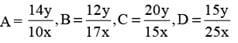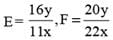So, E product has the highest price per unit.Use Code STAYHOME200 and get INR 200 additional OFF Use Coupon Code